# Derivatives as dy/dx

Derivatives are all about change ...

... they show how fast something is changing (called the rate of change) at any point.

In Introduction to Derivatives (please read it first!) we looked at how to do a derivative using differences and limits.

Here we look at doing the same thing but using the "dy/dx" notation (also called Leibniz's notation) instead of limits.

We start by calling the function "y":

y = f(x)

If x increases by Δx, then y increases by Δy

y + Δy = f(x + Δx)

## 2. Subtract the Two Formulas

 From: y + Δy = f(x + Δx) Subtract: y = f(x) To Get: y + Δy - y = f(x + Δx) - f(x) Simplify: Δy = f(x + Δx) - f(x)

## 3. Rate of Change

To work out how fast (called the rate of change) we divide by Δx: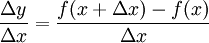## 4. Reduce Δx close to 0

We can't let Δx become 0 (because that would be dividing by 0), but we can make it very small, and call it "dx":

Δx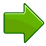dx

You can think of "dx" as being infinitesimal, or infinitely small.

Likewise Δy becomes very small and we call it "dy", to give us: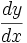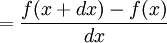## Try It On A Function

Let's try f(x) = x2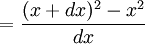f(x) = x2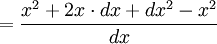Expand (x+dx)2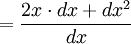Simplify (x2−x2=0)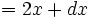Simplify fraction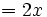dx goes (infinitely small)